Next: Generalized Momenta Up: Lagrangian Dynamics Previous: Atwood Machines

Sliding down a Sliding Plane

Consider the case of a particle of mass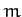sliding down a smooth inclined plane of mass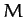which is, itself, free to slide on a smooth horizontal surface, as shown in Figure 34. This is a two degree of freedom system, so we need two coordinates to specify the configuration. Let us choose, the horizontal distance of the plane from some reference point, and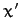, the parallel displacement of the particle from some reference point on the plane.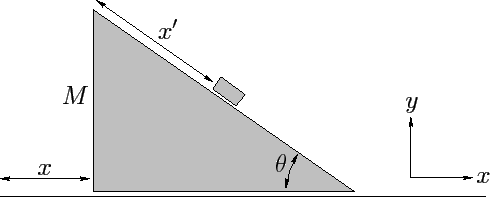Defining- and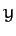-axes, as shown in the diagram, the- and-components of the particle's velocity are clearly given by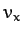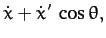(637)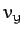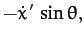(638)

respectively, whereis the angle of inclination of the plane with respect to the horizontal. Thus,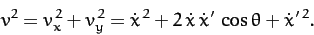(639)

Hence, the kinetic energy of the system takes the form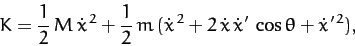(640)

whereas the potential energy is given by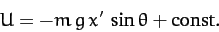(641)

It follows that the Lagrangian is written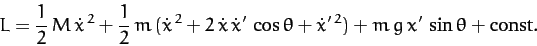(642)

The equations of motion,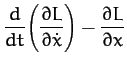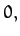(643)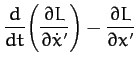(644)

thus yield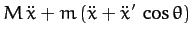(645)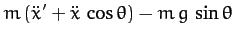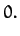(646)

Finally, solving for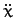and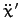, we obtain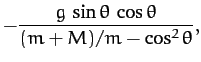(647)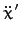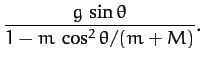(648)Next: Generalized Momenta Up: Lagrangian Dynamics Previous: Atwood Machines
Richard Fitzpatrick 2011-03-31